# The Schrdinger Wave Equation n The Schrdinger wave

• Slides: 29The Schrödinger Wave Equation n The Schrödinger wave equation in its time-dependent form for a particle of energy E moving in a potential V in one dimension is n The solution (wave function) is not restricted to being real. Only the physically measurable quantities must be real. These include the probability, momentum and energy. 1Normalization and Probability n The probability P(x) dx of a particle being between x and X + dx was given in the equation n The probability of the particle being between x 1 and x 2 is • The wave function must also be normalized so that the probability of the particle being somewhere is 1. Properties of Valid Wave Functions 1) 2) Normalizable Continuous with its 1 st and second derivatives (except for domains of null measure) 3) 2Stationary State n If the potential is time independent, the wave function can be written as: n The probability density becomes: n The probability distributions are constant in time. This is a stationary state. 3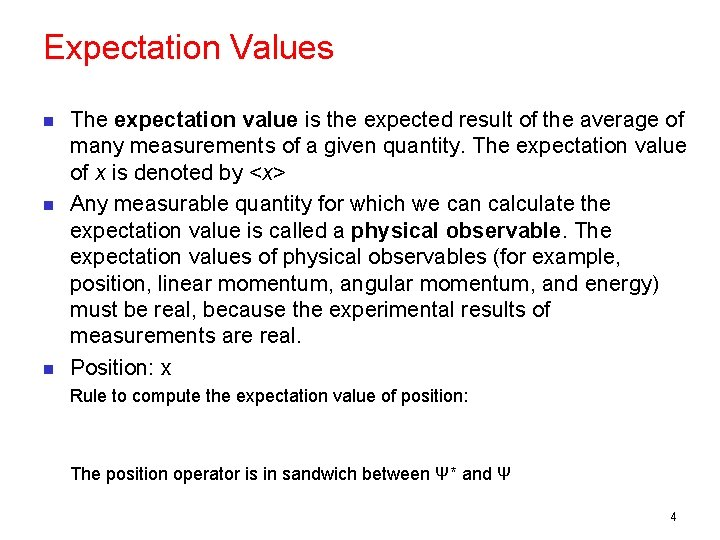Expectation Values n n n The expectation value is the expected result of the average of many measurements of a given quantity. The expectation value of x is denoted by <x> Any measurable quantity for which we can calculate the expectation value is called a physical observable. The expectation values of physical observables (for example, position, linear momentum, angular momentum, and energy) must be real, because the experimental results of measurements are real. Position: x Rule to compute the expectation value of position: The position operator is in sandwich between Ψ* and Ψ 4Momentum Operator n To find the expectation value of p, we first need to represent p in terms of x and t. Consider the derivative of the wave function of a free particle with respect to x: With k = p / ħ we have This yields n This suggests we define the momentum operator as n The expectation value of the momentum is . 5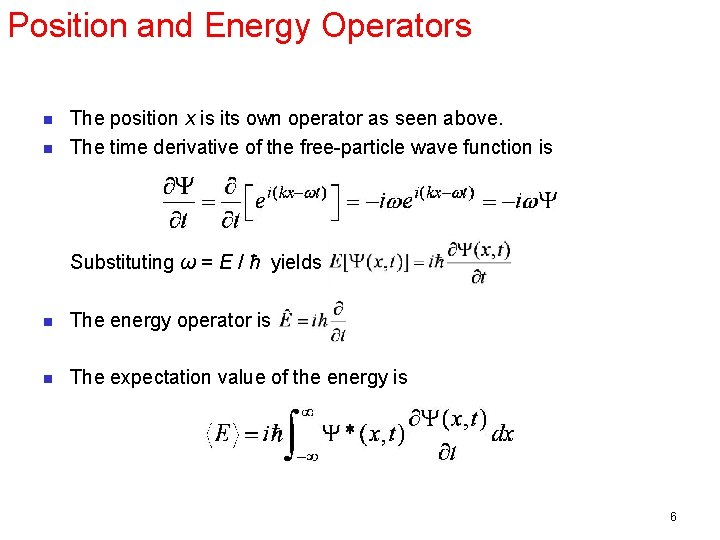Position and Energy Operators n n The position x is its own operator as seen above. The time derivative of the free-particle wave function is Substituting ω = E / ħ yields n The energy operator is n The expectation value of the energy is 6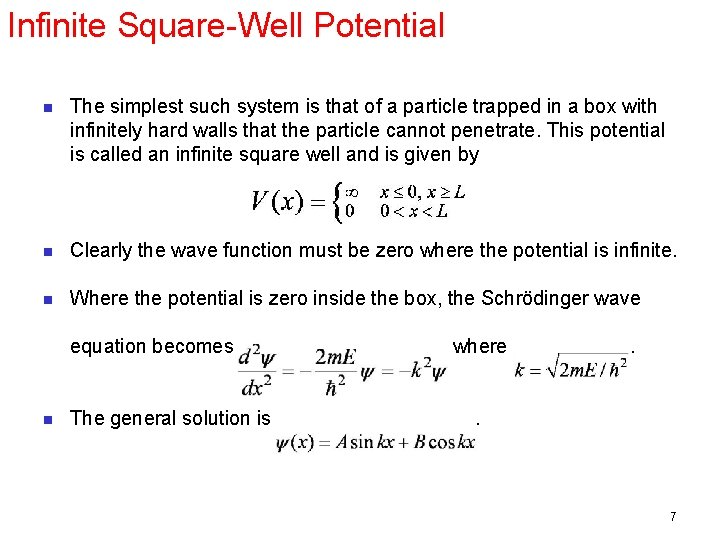Infinite Square-Well Potential n The simplest such system is that of a particle trapped in a box with infinitely hard walls that the particle cannot penetrate. This potential is called an infinite square well and is given by n Clearly the wave function must be zero where the potential is infinite. n Where the potential is zero inside the box, the Schrödinger wave equation becomes n The general solution is where . . 7Quantization n Boundary conditions of the potential dictate that the wave function must be zero at x = 0 and x = L. This yields valid solutions for integer values of n such that k. L = nπ. n The wave function is now n We normalize the wave function n The normalized wave function becomes n These functions are identical to those obtained for a vibrating string with fixed ends. 8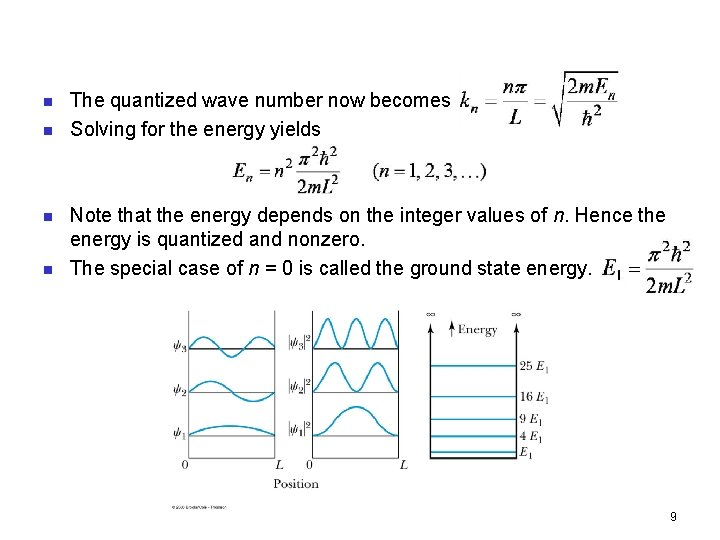n n The quantized wave number now becomes Solving for the energy yields Note that the energy depends on the integer values of n. Hence the energy is quantized and nonzero. The special case of n = 0 is called the ground state energy. 9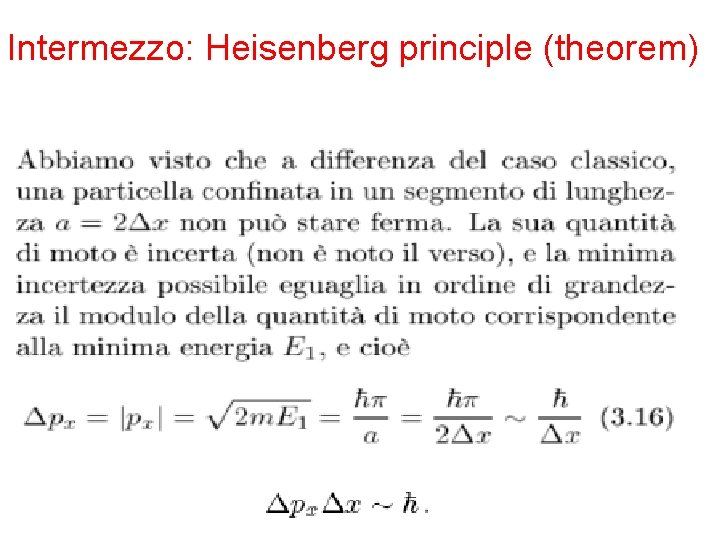Intermezzo: Heisenberg principle (theorem) 10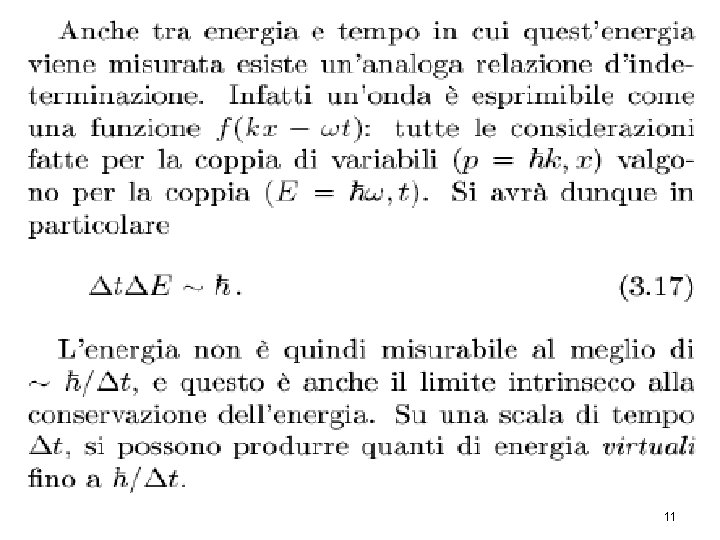11The uncertainty principle (qualitative) x(m) n n n Imagine that you're holding one end of a long rope, and you generate a wave by shaking it up and down rhythmically. Where is that wave? Nowhere, precisely - spread out over 50 m or so. What is its wavelength? It looks like ~6 m x(m) n By contrast, if you gave the rope a sudden jerk you'd get a relatively narrow bump traveling down the line. This time the first question (Where precisely is the wave? ) is a sensible one, and the second (What is its wavelength? ) seems difficult - it isn't even vaguely periodic, so how can you assign a wavelength to it? => Uncertainty is a characteristic of the wave representation 12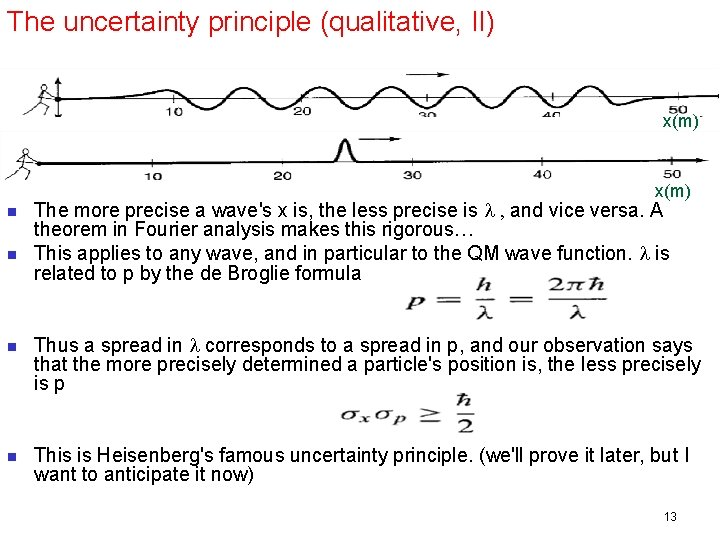The uncertainty principle (qualitative, II) x(m) n n The more precise a wave's x is, the less precise is l , and vice versa. A theorem in Fourier analysis makes this rigorous… This applies to any wave, and in particular to the QM wave function. l is related to p by the de Broglie formula Thus a spread in l corresponds to a spread in p, and our observation says that the more precisely determined a particle's position is, the less precisely is p This is Heisenberg's famous uncertainty principle. (we'll prove it later, but I want to anticipate it now) 13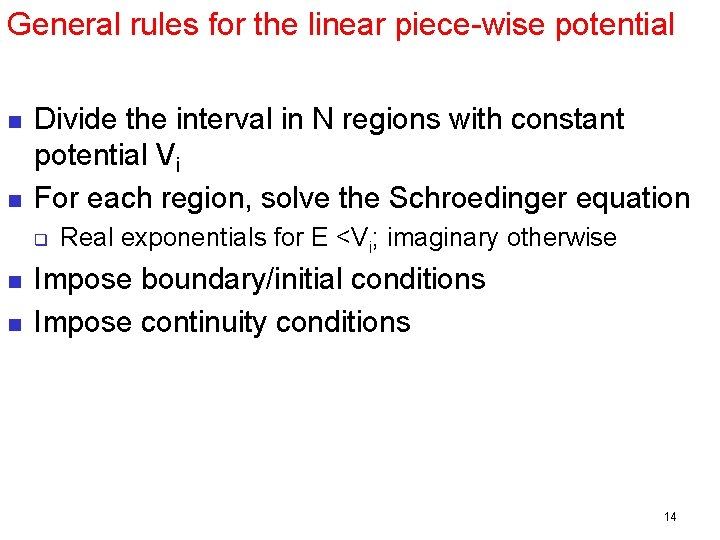General rules for the linear piece-wise potential n n Divide the interval in N regions with constant potential Vi For each region, solve the Schroedinger equation q n n Real exponentials for E <Vi; imaginary otherwise Impose boundary/initial conditions Impose continuity conditions 1415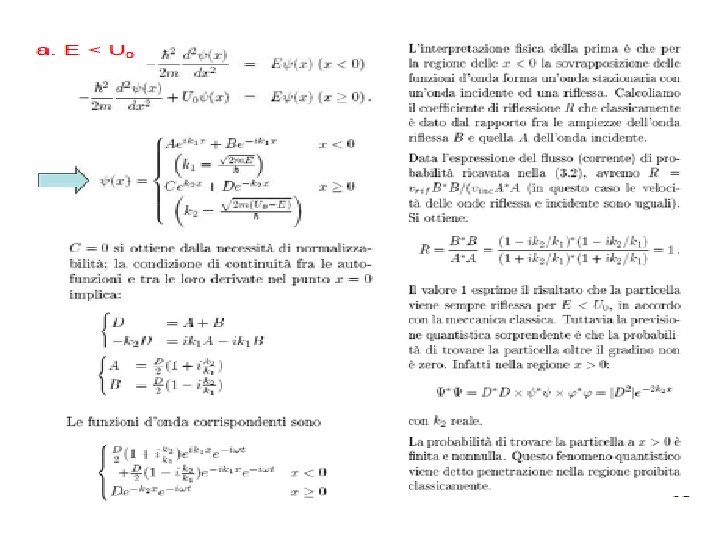16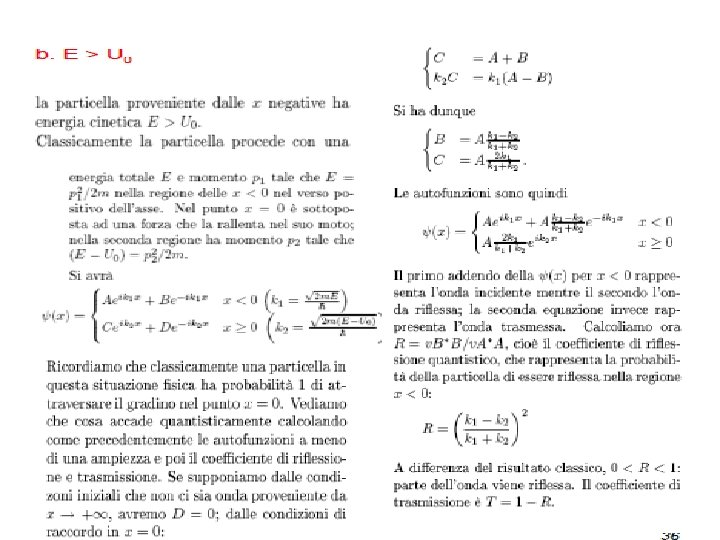17Finite Square-Well Potential n The finite square-well potential is n The Schrödinger equation outside the finite well in regions I and III is or using yields . Considering that the wave function must be zero at infinity, the solutions for this equation are 18Finite Square-Well Solution n Inside the square well, where the potential V is zero, the wave equation becomes where n Instead of a sinusoidal solution we have n The boundary conditions require that and the wave function must be smooth where the regions meet. n Note that the wave function is nonzero outside of the box. 19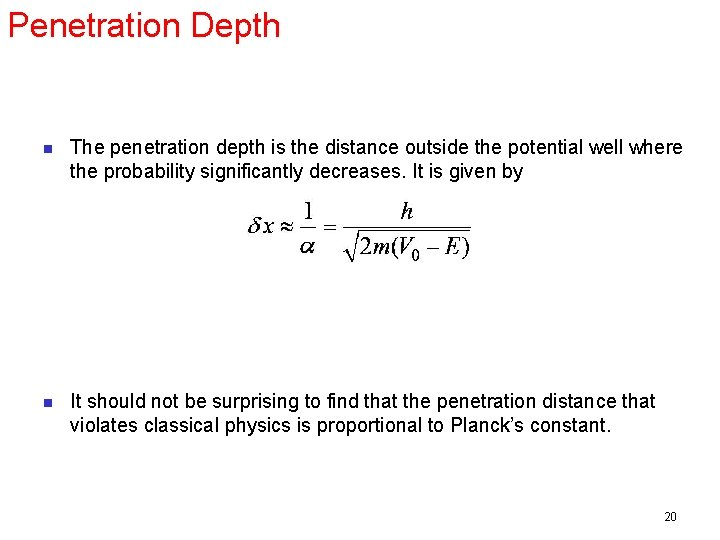Penetration Depth n The penetration depth is the distance outside the potential well where the probability significantly decreases. It is given by n It should not be surprising to find that the penetration distance that violates classical physics is proportional to Planck’s constant. 20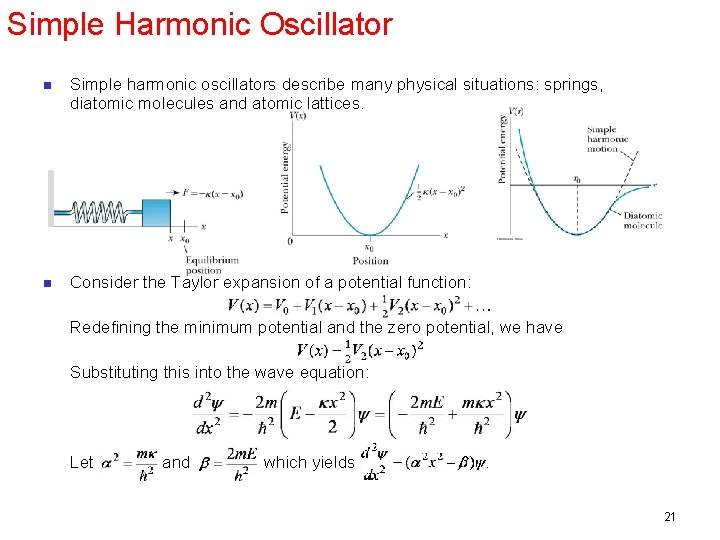Simple Harmonic Oscillator n Simple harmonic oscillators describe many physical situations: springs, diatomic molecules and atomic lattices. n Consider the Taylor expansion of a potential function: Redefining the minimum potential and the zero potential, we have Substituting this into the wave equation: Let and which yields . 21Parabolic Potential Well n n n If the lowest energy level is zero, this violates the uncertainty principle. The wave function solutions are where Hn(x) are Hermite polynomials of order n. In contrast to the particle in a box, where the oscillatory wave function is a sinusoidal curve, in this case the oscillatory behavior is due to the polynomial, which dominates at small x. The exponential tail is provided by the Gaussian function, which dominates at large x. 22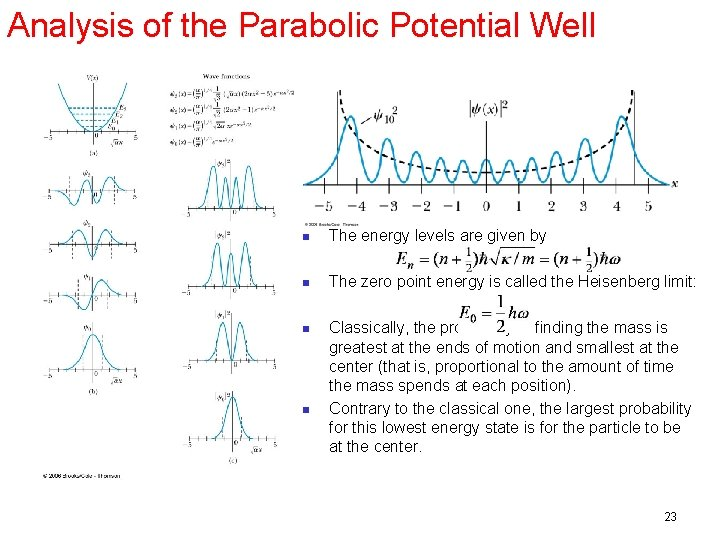Analysis of the Parabolic Potential Well n The energy levels are given by n The zero point energy is called the Heisenberg limit: n Classically, the probability of finding the mass is greatest at the ends of motion and smallest at the center (that is, proportional to the amount of time the mass spends at each position). Contrary to the classical one, the largest probability for this lowest energy state is for the particle to be at the center. n 23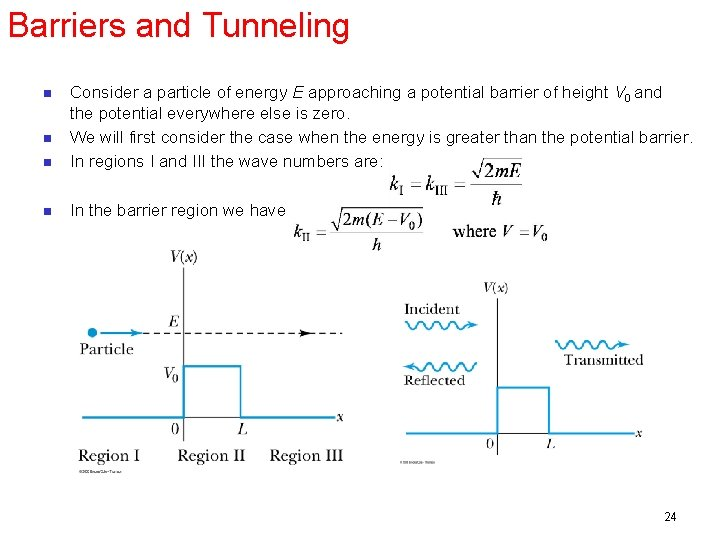Barriers and Tunneling n Consider a particle of energy E approaching a potential barrier of height V 0 and the potential everywhere else is zero. We will first consider the case when the energy is greater than the potential barrier. In regions I and III the wave numbers are: n In the barrier region we have n n 24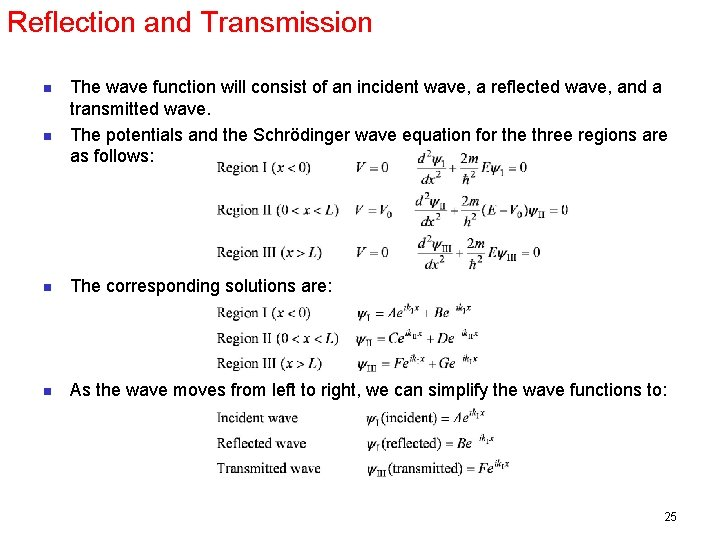Reflection and Transmission n n The wave function will consist of an incident wave, a reflected wave, and a transmitted wave. The potentials and the Schrödinger wave equation for the three regions are as follows: n The corresponding solutions are: n As the wave moves from left to right, we can simplify the wave functions to: 25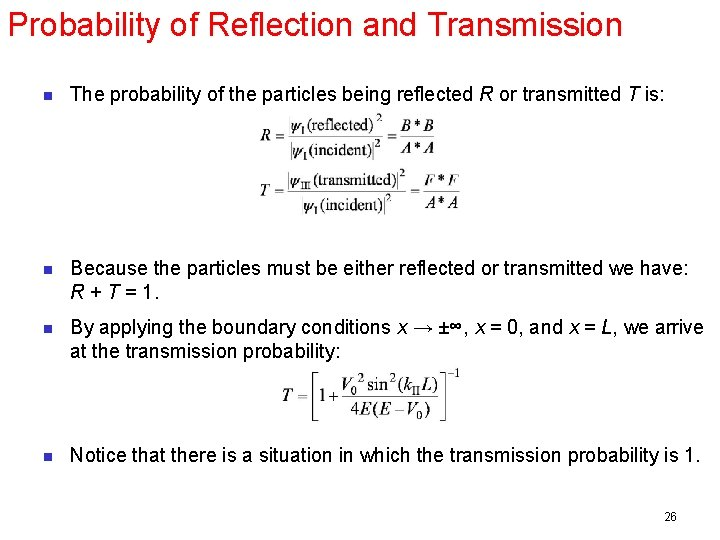Probability of Reflection and Transmission n The probability of the particles being reflected R or transmitted T is: n Because the particles must be either reflected or transmitted we have: R + T = 1. n By applying the boundary conditions x → ±∞, x = 0, and x = L, we arrive at the transmission probability: n Notice that there is a situation in which the transmission probability is 1. 26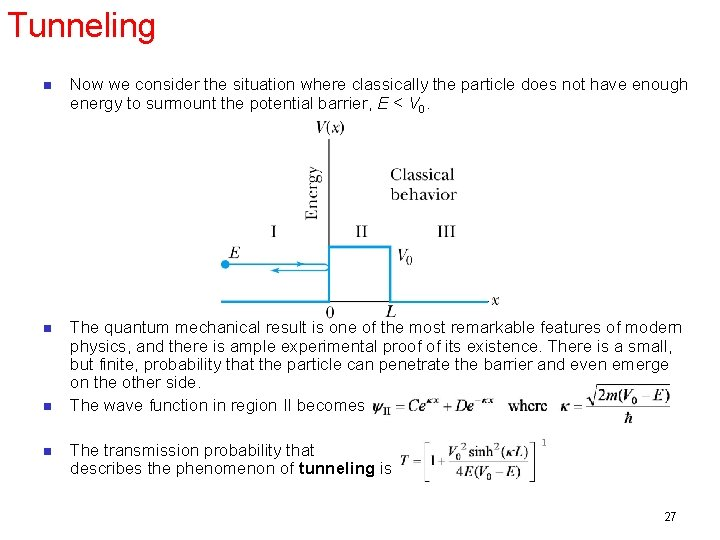Tunneling n Now we consider the situation where classically the particle does not have enough energy to surmount the potential barrier, E < V 0. n The quantum mechanical result is one of the most remarkable features of modern physics, and there is ample experimental proof of its existence. There is a small, but finite, probability that the particle can penetrate the barrier and even emerge on the other side. The wave function in region II becomes n n The transmission probability that describes the phenomenon of tunneling is 27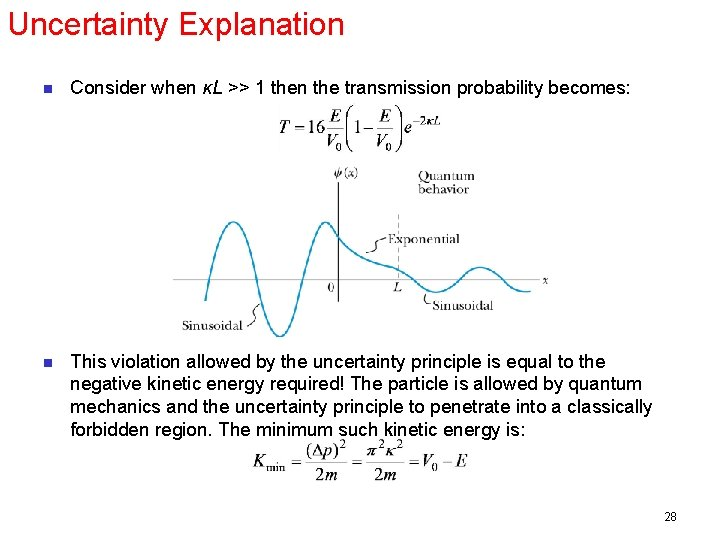Uncertainty Explanation n Consider when κL >> 1 then the transmission probability becomes: n This violation allowed by the uncertainty principle is equal to the negative kinetic energy required! The particle is allowed by quantum mechanics and the uncertainty principle to penetrate into a classically forbidden region. The minimum such kinetic energy is: 28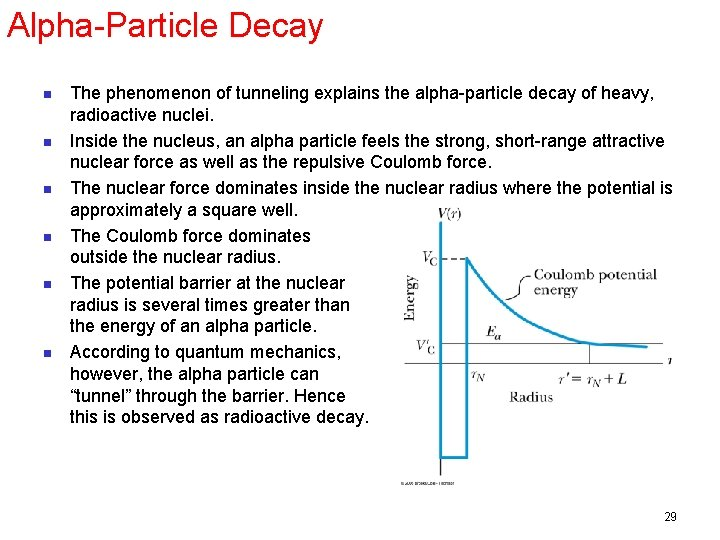Alpha-Particle Decay n n n The phenomenon of tunneling explains the alpha-particle decay of heavy, radioactive nuclei. Inside the nucleus, an alpha particle feels the strong, short-range attractive nuclear force as well as the repulsive Coulomb force. The nuclear force dominates inside the nuclear radius where the potential is approximately a square well. The Coulomb force dominates outside the nuclear radius. The potential barrier at the nuclear radius is several times greater than the energy of an alpha particle. According to quantum mechanics, however, the alpha particle can “tunnel” through the barrier. Hence this is observed as radioactive decay. 29